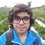# Pre-RMO 2014/7

If $x^{(x^4)} = 4$, what is the value of $x^{(x^2)} + x^{(x^8)}$?

This note is part of the set Pre-RMO 2014Note by Pranshu Gaba
6 years, 8 months ago

This discussion board is a place to discuss our Daily Challenges and the math and science related to those challenges. Explanations are more than just a solution — they should explain the steps and thinking strategies that you used to obtain the solution. Comments should further the discussion of math and science.

When posting on Brilliant:

• Use the emojis to react to an explanation, whether you're congratulating a job well done , or just really confused .
• Ask specific questions about the challenge or the steps in somebody's explanation. Well-posed questions can add a lot to the discussion, but posting "I don't understand!" doesn't help anyone.
• Try to contribute something new to the discussion, whether it is an extension, generalization or other idea related to the challenge.
• Stay on topic — we're all here to learn more about math and science, not to hear about your favorite get-rich-quick scheme or current world events.

MarkdownAppears as
*italics* or _italics_ italics
**bold** or __bold__ bold
- bulleted- list
• bulleted
• list
1. numbered2. list
1. numbered
2. list
Note: you must add a full line of space before and after lists for them to show up correctly
paragraph 1paragraph 2

paragraph 1

paragraph 2

[example link](https://brilliant.org)example link
> This is a quote
This is a quote
    # I indented these lines
# 4 spaces, and now they show
# up as a code block.

print "hello world"
# I indented these lines
# 4 spaces, and now they show
# up as a code block.

print "hello world"
MathAppears as
Remember to wrap math in $$ ... $$ or $ ... $ to ensure proper formatting.
2 \times 3 $2 \times 3$
2^{34} $2^{34}$
a_{i-1} $a_{i-1}$
\frac{2}{3} $\frac{2}{3}$
\sqrt{2} $\sqrt{2}$
\sum_{i=1}^3 $\sum_{i=1}^3$
\sin \theta $\sin \theta$
\boxed{123} $\boxed{123}$

## Comments

Sort by:

Top Newest

Let $x=y^{\frac{1}{4}}$

$(\sqrt{y})^{y}=4$

$y^{y}=4^{4}$

Comparing both sides we get $y=4 \Rightarrow x=\sqrt{2}$

Pluging the value of x in the expression we get $\boxed{258}$

Note: I like keeping it real (Its a joke)

- 6 years, 8 months ago

Log in to reply

The equation $x^{x^{4}}$ have 4 roots

$\pm \sqrt2, \pm i \sqrt2$

It should be mentioned in the question that x is real because answer can vary

- 6 years, 8 months ago

Log in to reply

Totally agree

- 6 years, 8 months ago

Log in to reply

Although this is right, such a comparison may not give all answers in some cases. e.g. $x^{1/x}=2^{1/2}$ suggests $x=2$ as a trivial answer, but observe that $x=4$ is also a nontrivial answer. One needs to be careful thus. Try to justify therefore that in the above case however, $y=4$ is the only real answer (It may be very tough to prove/disprove that there may be an imaginary solution to this.)

- 6 years, 7 months ago

Log in to reply

The function $\sqrt[x]{x}=y$ always gives 2 real solutions for $y\leq e$, but function $x^x=y$ gives 2 real values only for $\frac{1}{e} \leq y \leq 1$ and since $4^4>1$ we have only one real solution.

- 6 years, 7 months ago

Log in to reply

Well although you got most of it right, it is not that easy to summarize it like this.

Firstly what you said about the function $x^{1/x}$ should be modified as $x^{1/x}=y$ always gives two positive real solutions for $x$ if $1. There may still be some negative solutions (Yes the function is defined for some subset of negative numbers, try to find it as an exercise).

Talking about $x^x=y$, it has only one positive solution in $x$ if $y\ge 1$. This equation may still have a negative solution in $x$. Why I'm saying here it may have a negative solution is because the solution may or may not exist and it may be very hard to prove existence. But in this question, we need not worry about the negative values of $y$ as otherwise $x$ would become complex and the question would not have a unique solution which will also go beyond the scope of the exam. For it to make sense in the exam point of view, we make an implicit assumption that $x$ is real.

- 6 years, 7 months ago

Log in to reply

The answer is 258. I'm still not clear on whether we just have to substitute the values in the actual paper or solve it using some other method.

- 6 years, 8 months ago

Log in to reply

$x = \sqrt2$

Ans 258

- 6 years, 8 months ago

Log in to reply

Can you tell which method did you use to get $x=\sqrt{2}$?

- 6 years, 8 months ago

Log in to reply

18

- 6 years, 8 months ago

Log in to reply

258

- 6 years, 1 month ago

Log in to reply

258

- 5 years, 10 months ago

Log in to reply

258

- 5 years, 10 months ago

Log in to reply

Can easily be solved by taking log

- 5 years ago

Log in to reply

How?

- 2 years, 10 months ago

Log in to reply

×

Problem Loading...

Note Loading...

Set Loading...